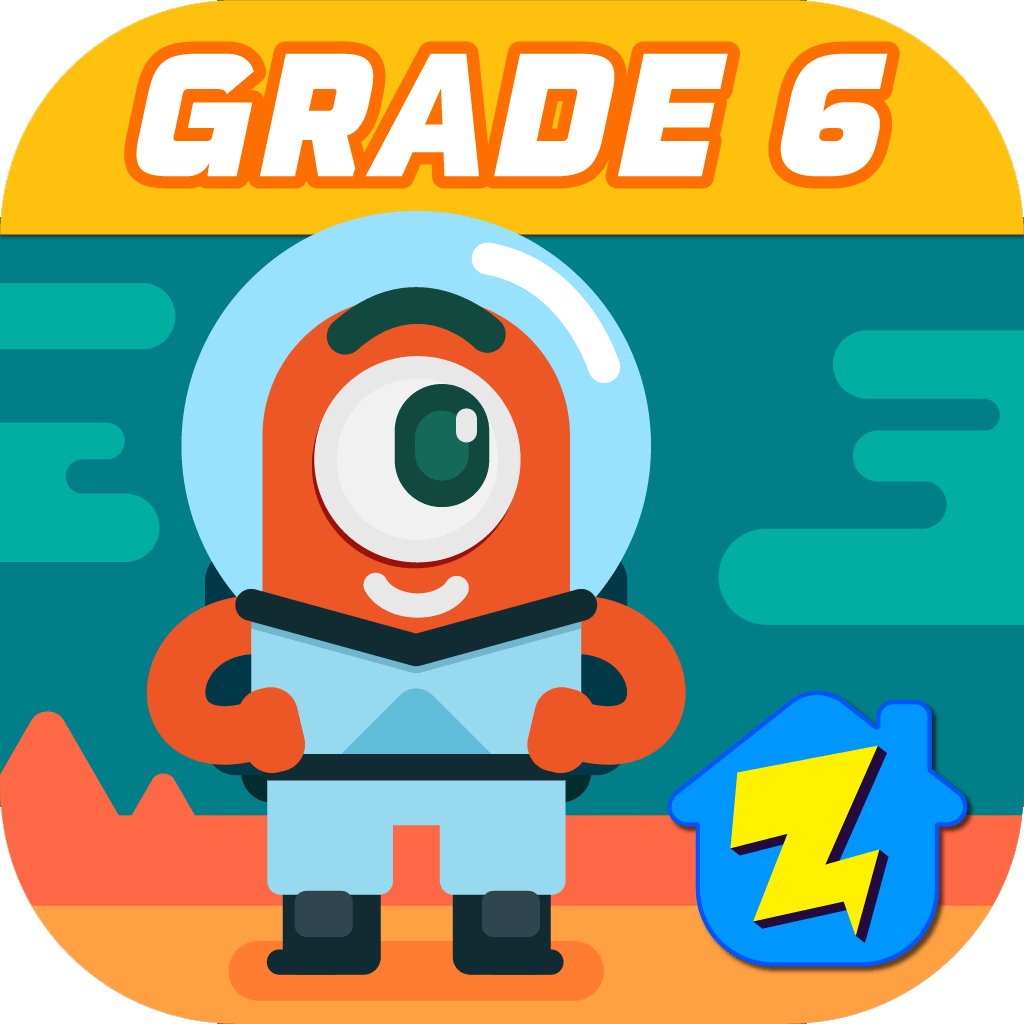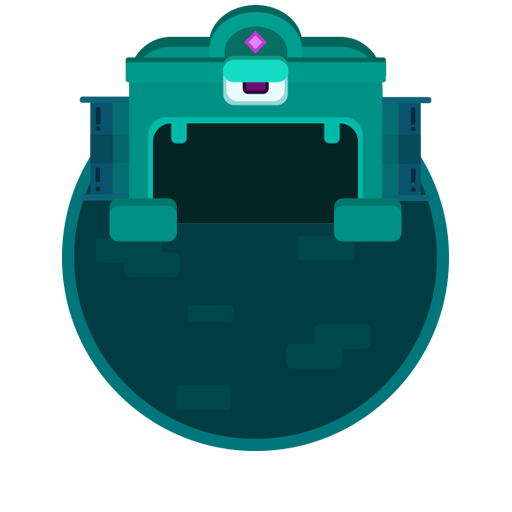## Welcome to Zapzapmath Grade 6 Home App### This app helps players learn about ratios, divide fractions by fractions, find the greatest common factor, solve equations and inequalities, and understand statistic variablity and distributions.

This section contains 6th grade math games which offer students to learn more about important key points in mathematics such as interpreting bar graphs, identifying the greatest common factor, and telling the median, mean, mode, and range. Sixth grade students who are introduced to these games will gain an extensive knowledge about topics of the significant math courses covered.

These 6th grade math games will provide students in the sixth grade with the best methods to use mathematical concepts such as median, mode, mean, range, and bar graphs as empirical evidences for data collection.

### Skills that can be learned

##### Data distribution of bar graphs

Interpreting the distribution of data sets of bar graphs

##### Greatest common factor

Knowing how to find the greatest common factor

##### Mode, median, mean, and range

Knowing the meanings of mode, median, mean, and range

##### Fractions to percentage

Converting fractions to percentage

### Games Available In This Grade### Fraction To Percentage : Hot Head

Convert fractions to percentage### Definition Of Greatest Common Factor : Burger Bar

Find the greatest common factor### Statistic And Probability : Ancient Enigma

Understand mean, median, and mode### Mode Median Mean And Range : Snakes and Ladders

Describe the observation of data### What Is A Bar Graph : Maze Escape

Identify distribution of data